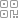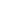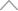6 0 8 4 5 1 9

深度学习中的轻量级网络架构总结与代码实现

导读安装使用

pip install light_cnns

项目目录MobileNets系列

MobileNetV1

MobileNets: Efficient Convolutional Neural Networks for Mobile Vision Applications

https://arxiv.org/abs/1704.04861• 代码实现

import torch

from light_cnns import mbv1

model = mbv1()

model.eval()

print(model)

input = torch.randn(13224224)

y = model(input)

print(y.size())

MobileNetv2

MobileNetV2: Inverted Residuals and Linear Bottlenecks

https://arxiv.org/abs/1704.04861

mobilenetv2 沿用特征复用结构（残差结构），首先进行Expansion操作然后再进行Projection操作，最终构建一个逆残差网络模块（即Inverted residual block）。

• 增强了梯度的传播，显著减少推理期间所需的内存占用。

• 使用 RELU6（最高输出为 6）激活函数，使得模型在低精度计算下具有更强的鲁棒性。

• 在经过projection layer转换到低维空间后，将第二个pointwise convolution后的 ReLU6改成Linear结构，保留了特征多样性，增强网络的表达能力（Linear Bottleneck）• 代码实现

import torch

from light_cnns import mbv2

model = mbv2()

model.eval()

print(model)

input = torch.randn(13224224)

y = model(input)

print(y.size())

MobileNetv3

Searching for MobileNetV3

https://arxiv.org/abs/1905.02244

• 网络的架构基于NAS实现的MnasNet（效果比MobileNetV2好）

• 论文推出两个版本：Large 和 Small，分别适用于不同的场景;

• 继承了MobileNetV1的深度可分离卷积

• 继承了MobileNetV2的具有线性瓶颈的倒残差结构

• 引入基于squeeze and excitation结构的轻量级注意力模型(SE)

• 使用了一种新的激活函数h-swish(x)• 代码实现

import torch

from light_cnns import mbv3_small

#from light_cnns import mbv3_large

model_small = mbv3_small()

#model_large = mbv3_large()

model_small.eval()

print(model_small)

input = torch.randn(13224224)

y = model_small(input)

print(y.size())

MobileNext

Rethinking Bottleneck Structure for Efficient Mobile Network Design

https://arxiv.org/abs/2007.02269• 代码实现

import torch

from light_cnns import mobilenext

model = mobilenext()

model.eval()

print(model)

input = torch.randn(13224224)

y = model(input)

print(y.size())

ShuffleNet系列

ShuffleNetv1

ShuffleNet: An Extremely Efficient Convolutional Neural Network for Mobile Devices

https://arxiv.org/abs/1707.01083• 代码实现

import torch

from light_cnns import shufflenetv1

model = shufflenetv1()

model.eval()

print(model)

input = torch.randn(13224224)

y = model(input)

print(y.size())

ShuffleNetv2

ShuffleNet V2: Practical Guidelines for Efficient CNN Architecture Design

https://arxiv.org/abs/1807.11164import torch

from light_cnns import shufflenetv2

model = shufflenetv2()

model.eval()

print(model)

input = torch.randn(13224224)

y = model(input)

print(y.size())

华为诺亚轻量级网络系列

AdderNet：Do We Really Need Multiplications in Deep Learning?import torch

from light_cnns import resnet20

model = resnet20()

model.eval()

print(model)

input = torch.randn(13224224)

y = model(input)

print(y.size())

GhostNet：More Features from Cheap Operations

https://arxiv.org/abs/1911.11907• 代码实现

import torch

from light_cnns import ghostnet

model = ghostnet()

model.eval()

print(model)

input = torch.randn(13224224)

y = model(input)

print(y.size())

轻量级注意力网络架构

Coordinate Attention for Efficient Mobile Network Design

https://arxiv.org/abs/2103.02907Code

import torch

from light_cnns import mbv2_ca

model = mbv2_ca()

model.eval()

print(model)

input = torch.randn(13224224)

y = model(input)

print(y.size())

新知精选

优选课程 新知学院

•扫码下载商业新知APP~

•回到顶部